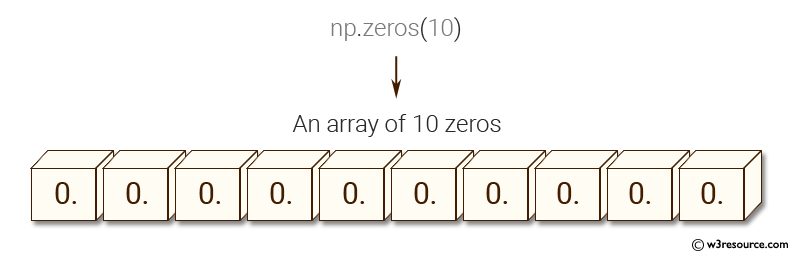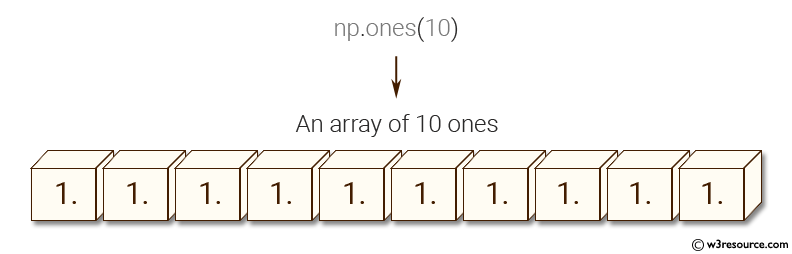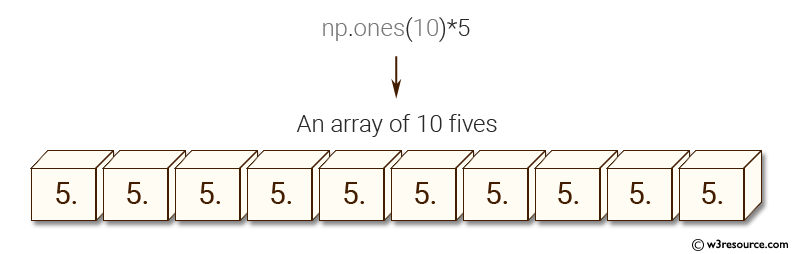﻿ NumPy: Create an array of 10 zeros, 10 ones, 10 fives - w3resource# NumPy: Create an array of 10 zeros, 10 ones, 10 fives

## NumPy: Basic Exercise-13 with Solution

Write a NumPy program to create an array of 10 zeros, 10 ones, 10 fives.

Sample Solution :

Python Code :

``````import numpy as np
array=np.zeros(10)
print("An array of 10 zeros:")
print(array)
array=np.ones(10)
print("An array of 10 ones:")
print(array)
array=np.ones(10)*5
print("An array of 10 fives:")
print(array)
``````

Sample Output:

```An array of 10 zeros:
[ 0.  0.  0.  0.  0.  0.  0.  0.  0.  0.]
An array of 10 ones:
[ 1.  1.  1.  1.  1.  1.  1.  1.  1.  1.]
An array of 10 fives:
[ 5.  5.  5.  5.  5.  5.  5.  5.  5.  5.]
```

Pictorial Presentation:Python Code Editor:

Have another way to solve this solution? Contribute your code (and comments) through Disqus.

What is the difficulty level of this exercise?

Test your Python skills with w3resource's quiz

﻿

## Python: Tips of the Day

Returns the symmetric difference between two lists, after applying the provided function to each list element of both

Example:

```def tips_symmetric_difference_by(p, q, fn):
_p, _q = set(map(fn, p)), set(map(fn, q))
return [item for item in p if fn(item) not in _q] + [item for item in q if fn(item) not in _p]
from math import floor
print(tips_symmetric_difference_by([4.2, 2.4], [4.6, 6.8],floor))
```

Output:

```[2.4, 6.8]
```